# Greatest common factor

Here you will learn about the greatest common factor, including a review of prime factorization and how to use it to find the greatest common factor.

Students will first learn about the greatest common factor as part of the number system in 6th grade.

## What is the greatest common factor?

The greatest common factor (GCF) is the largest integer that two or more numbers can both be divided by. The name itself tells you what the skill is:

For example,

Find the greatest common factor of 4 and 6.

By listing the factors of 4 and the factors of 6, you can see that the greatest number that occurs in each list is 2, so the greatest common factor of 4 and 6 is 2.

You may have noticed that 1 occurs in both lists. This is a common factor, but not the greatest common factor.

### The Prime Factorization Method

Calculating the greatest common factor becomes more complicated for larger numbers. Listing all the factors of each number can be time consuming and it is easy to forget a factor.

To make it simpler, you can use the prime factors of both numbers. You can use prime factors and a Venn diagram to calculate the Greatest Common Factor.

You need to write out the prime factorization of each number fully and then put the numbers into the Venn diagram by looking for pairs.

Here, you have the prime factors of 12 and 30.

• The yellow section represents the prime factors of 12 that are not prime factors of 30 (which is just the value 2 ).
• The blue section represents the prime factors of 30 that are not prime factors of 12 (which is just the value 5 ).
• The green intersection represents the shared prime factors of 12 and 30. Here, the prime factors of 2 and 3 occur for both 12 and 30. Their product is the greatest common factor.

The greatest common factor for 12 and 30 is 2 \times 3 = 6.

### What is the greatest common factor?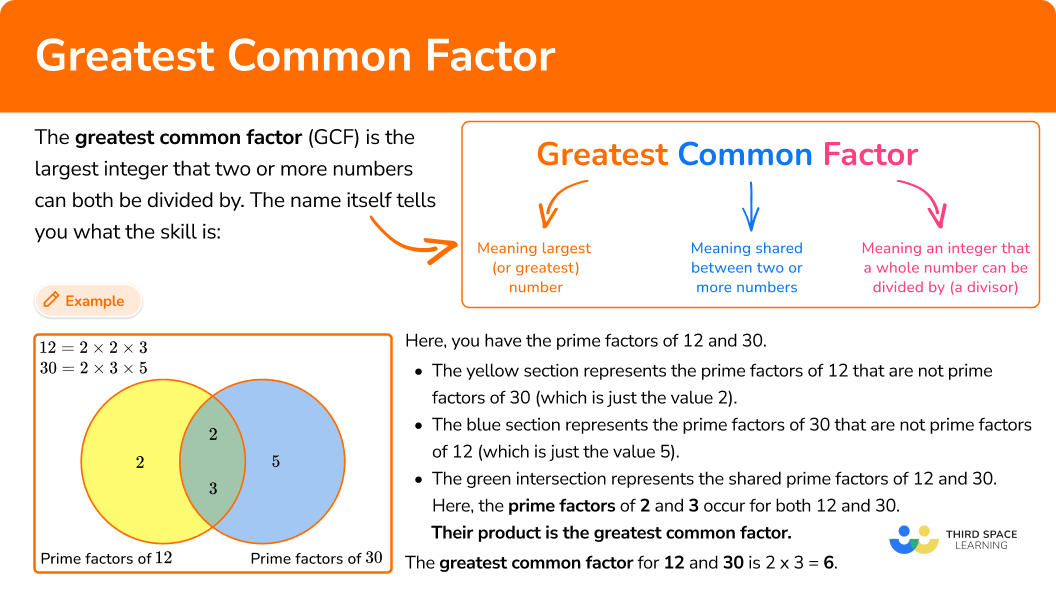## Common Core State Standards

How does this relate to 6th grade math?

• Grade 6 – The Number System (6.NS.B.4)
Find the greatest common factor of two whole numbers less than or equal to 100 and the least common multiple of two whole numbers less than or equal to 12. Use the distributive property to express a sum of two whole numbers 1–100 with a common factor as a multiple of a sum of two whole numbers with no common factor. For example, express 36 + 8 as 4 (9 + 2).

## How to calculate the greatest common factor (GCF)

In order to calculate the greatest common factor for two or more numbers:

1. State the product of prime factors for each number.
2. Write all the prime factors into the Venn diagram for each number.
3. Multiply the prime factors in the intersection to find the GCF.

## Greatest common factor examples

### Example 1: Two simple composite numbers

Calculate the greatest common factor of 18 and 24.

1. State the product of prime factors for each number.

\begin{aligned} & 18=2 \times 3 \times 3 \\\\ & 24=2 \times 2 \times 2 \times 3 \end{aligned}

2Write all the prime factors into the Venn diagram for each number.

3Multiply the prime factors in the intersection to find the GCF.

GCF = 2 \times 3=6

### Example 2: One composite number is a factor of the other

Calculate the greatest common factor of 14 and 56.

\begin{aligned} & 14=2 \times 7 \\\\ & 56=2 \times 2 \times 2 \times 7 \end{aligned}

GCF =2 \times 7=14

### Example 3: Calculate the prime factorization first

Given that 90=2 \times 3^2 \times 5, calculate the greatest common factor of 54 and 90.

To state the product of prime factors of 54, you use a factor tree:

\begin{aligned} & 54=2 \times 3 \times 3 \times 3 \\\\ & 90=2 \times 3 \times 3 \times 5 \end{aligned}

GCF =2 \times 3 \times 3=18

### Example 4: Three composite numbers

Calculate the greatest common factor of 12, 20, and 32.

\begin{aligned} & 12=2 \times 2 \times 3 \\\\ & 20=2 \times 2 \times 5 \\\\ & 32=2 \times 2 \times 2 \times 2 \times 2 \end{aligned}

GCF =2 \times 2=4

### Example 5: Only common factor is 1

Calculate the greatest common factor of 35 and 72.

\begin{aligned} & 35=5 \times 7 \\\\ & 72=2 \times 2 \times 2 \times 3 \times 3 \end{aligned}

Here there are no prime factors in the intersection, so the greatest common factor is 1.

### Example 6: Word problem

Sue sells apples in baskets. On Thursday, she expects to receive a delivery of 36 apples in the morning and 42 apples in the afternoon. Sue wants to divide each crate equally into smaller baskets, so she has the same number of apples in each basket.

What is the maximum number of apples she can put in each basket so that they contain the same quantity during the whole day?

\begin{aligned} & 36=2 \times 2 \times 3 \times 3 \\\\ & 42=2 \times 3 \times 7 \end{aligned}

GCF =2 \times 3=6

To answer the question: She should put 6 apples in each basket.

### Teaching tips for greatest common factor

• Start with small numbers, so students can focus on understanding and learning the procedure. Then, move to large numbers to challenge students’ ability to find all the prime factors of a number.

• Instead of relying only on worksheets, look for opportunities for students to solve real-world problems that involve GCF.

• Some students may struggle to find all the prime factors, especially for larger numbers. You may provide a calculator to assist students so that they can focus on the skill, not on the calculations.

### Our favorite mistakes

• Calculating the least common multiple (LCM) instead of GCF
It is common to mix up the greatest common factor with the least common multiple. To avoid this, remember the definition of factors and multiples.
Factor: the number being multiplied
Multiple: the product of two or more factors being multiplied
For example,
What is the GCF of 2 and 10? What is the LCM of 2 and 10?
Factors of 2\text{:} \; 1, 2 Multiples of 2\text{:} \; 2, 4, 6, 8, 10…
Factors of 10\text{:}\; 1, 2, 5, 10 Multiples of 10\text{:}\; 10, 20, 30…
The GCF is 2 The LCM is 10

• Not writing duplicate factors in the Venn diagram
It is possible to forget to include prime factors in a Venn diagram when the factors are repeated.
For example,
What is the GCF of 54 and 81?

### Greatest common factor practice questions

1. Calculate the greatest common factor of 22 and 60.

1660112\begin{aligned} &22= 2 \times 11\\\\ &60=2 \times 2 \times 3 \times 5 \end{aligned}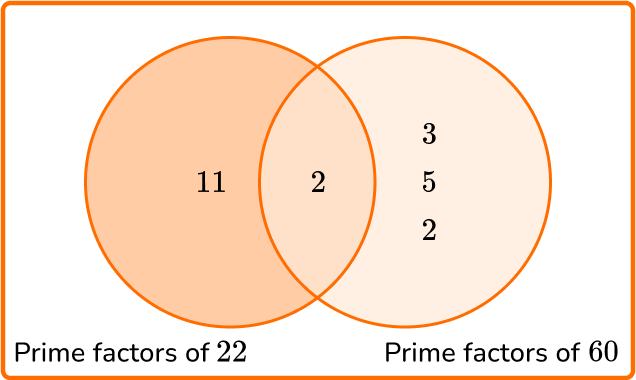GCF = 2

2. Calculate the greatest common factor of 21 and 63.

372163\begin{aligned} &21=3 \times 7\\\\ &63= 3 \times 3 \times 7 \end{aligned}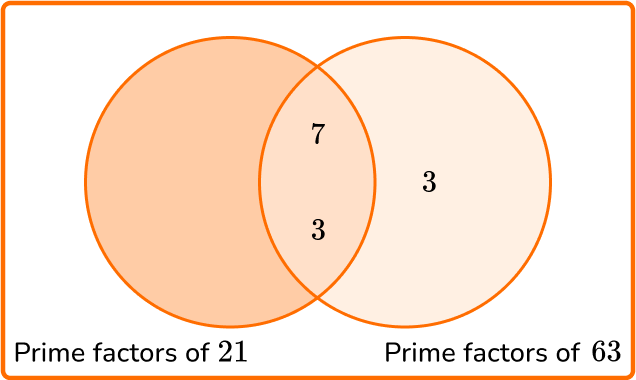GCF =7 \times 3=21

3. Calculate the greatest common factor of 90 and 135.

45151270\begin{aligned} &90= 2 \times 3 \times 3 \times 5\\\\ &135=3 \times 3 \times 3 \times 5 \end{aligned}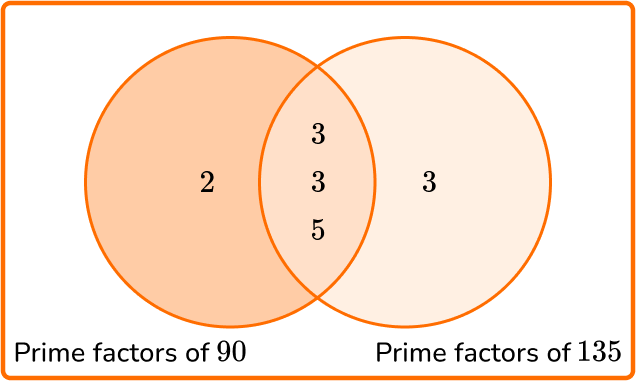GCF =3\times 3 \times 5=45

4. Calculate the greatest common factor of 12, 30, and 48.

12236\begin{aligned} &12=2 \times 2 \times 3\\\\ &30=2 \times 3 \times 5\\\\ &48=2 \times 2 \times 2 \times 2 \times 3 \end{aligned}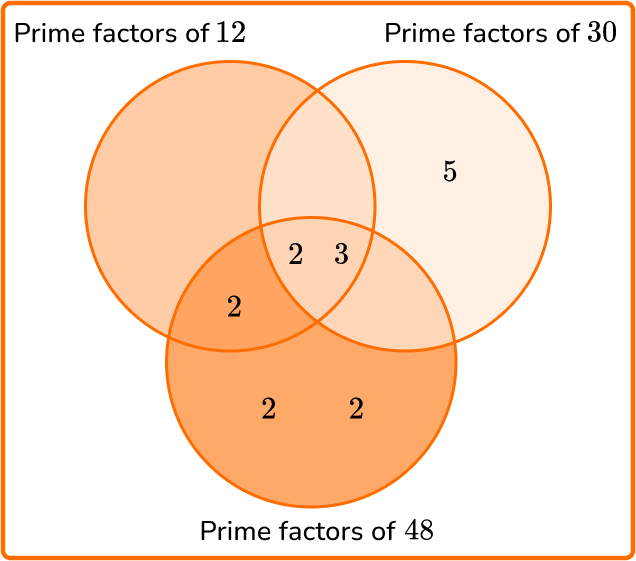GCF = 2 \times 3=6

5. Calculate the greatest common factor of 21 and 58.

0131218\begin{aligned} &21=3 \times 7\\\\ &58= 2 \times 29 \end{aligned}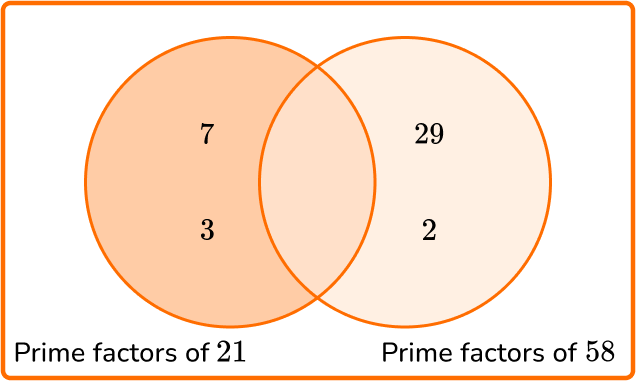All numbers have a factor of 1.

GCF = 1

6. Josh is grouping 3 different colored pens. He has 18 blue, 16 black, and 28 red pens.

Each group must contain the same number of blue pens, the same number of black pens, and the same number of red pens. How many groups can Josh make so that every pen is in a group?

2141,008Calculate the GCF of 18, 16, and 28.

\begin{aligned} &18=2 \times 3 \times 3\\\\ &16=2 \times 2 \times 2 \times 2\\\\ &28=2 \times 2 \times 7 \end{aligned}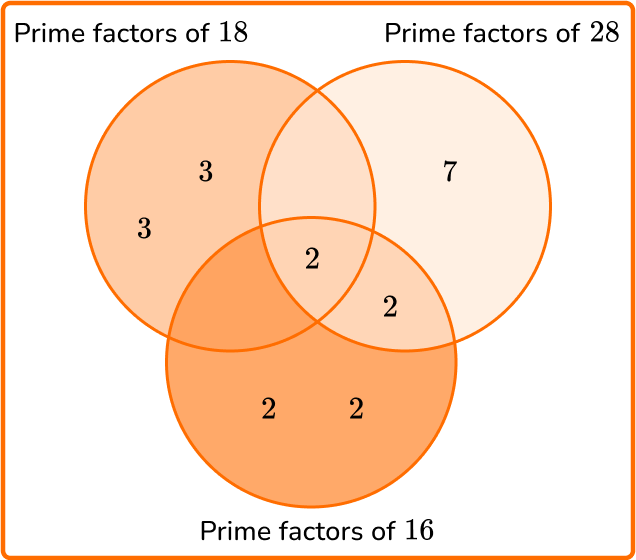GCF = 2

Therefore Josh can make 2 groups of pens with the same number of each color in each group.

## Greatest common factor FAQs

Why does the intersection of the Venn diagram contain the GCF?

The intersection contains the greatest common factor because all the shared prime factors are in the intersection. There are no other common prime factors in the set of numbers, otherwise, they would be in the intersection.

Are there other terms for Greatest Common Factor (GCF)?

Greatest Common Divisor (GCD), Greatest Common Denominator (GCD), and Highest Common Factor (HCF) are other terms that mean the same as Greatest Common Factor.

Does the largest number always have more prime factors?

No, not necessarily. For example, the number 24=2 \times 2 \times 2 \times 3 has 4 prime factors while 41=41 \times 1 has only 1, so in this case the smallest number has more factors.

Is the greatest factor always the GCF?

No, not necessarily. In order to be the GCF, it has to be a factor of all the given numbers. If it is not a common factor of all the numbers, it is not the GCF.

## Still stuck?

At Third Space Learning, we specialize in helping teachers and school leaders to provide personalized math support for more of their students through high-quality, online one-on-one math tutoring delivered by subject experts.

Each week, our tutors support thousands of students who are at risk of not meeting their grade-level expectations, and help accelerate their progress and boost their confidence.